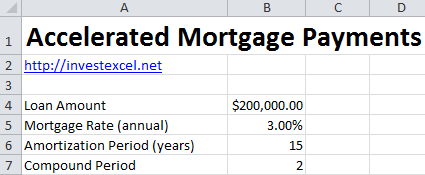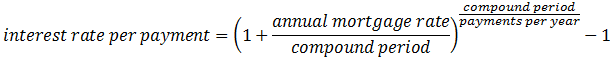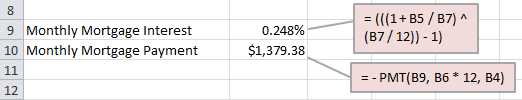# How to Calculate Accelerated Mortgage Payments in Excel

Accelerated mortgage payments can save you thousands of dollars in interest. They’re rapidly becoming the tool of choice for frugal homeowners.

By increasing the total amount you pay over a year, you pay less interest across the life of the mortgage.

Banks commonly offer two accelerated schemes.

Accelerated weekly – this divides your monthly payment into four. Because there are more than four weeks in a month, you effectively make two extra payment every year.

Accelerated bi-weekly – this divides your monthly payment into two equal parts. And because there are more than four weeks in a month, you effectively make an extra payment every year.

Imagine you’re a savvy mortgage shopper and you want to calculate payments for an accelerated scheme. You’d start by cracking open Excel, and entering your

• loan amount,
• mortgage rate,
• amortization period,
• and interest compounding period (this is 12 for US mortgages, 2 for Canadian mortgages, and 1 for UK mortgages).

Your spreadsheet might look like this.Then you calculate the monthly mortgage interest and payment.

The monthly mortgage interest is given by this formula.The monthly payment is given by Excel’s PMT function. You give PMT three arguments:

• the interest rate per payment
• the number of payments (in this case it would be the amortization period in years multiplied by 12)
• and the loan amount

At this point, your spreadsheet might look like this.And then finally, you calculate the accelerated payments. You’ll simply divide the monthly payment in two and four respectively for accelerated bi-weekly and weekly payments. Excel makes this easy.

Get the complete spreadsheet at the link below. You may also want to get the Mortgage Payment Calculator. This free spreadsheet is a complete solution for calculating your payments. It also gives you a table of payment dates, so you can budget appropriately.

Get Excel Spreadsheet to Calculate Accelerated Mortgage Payments